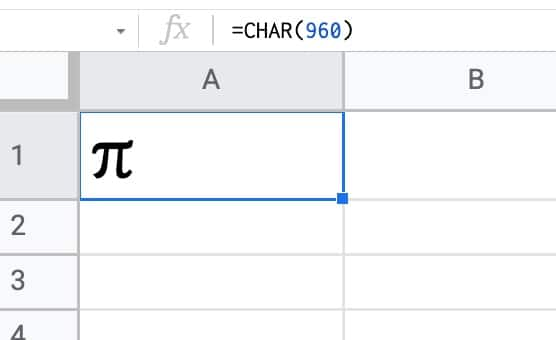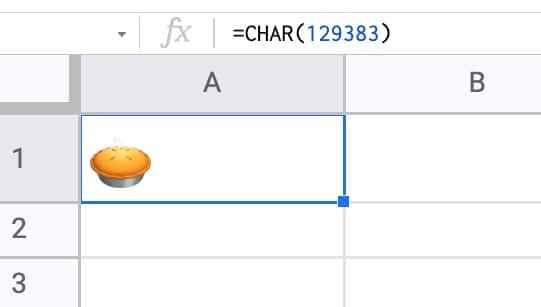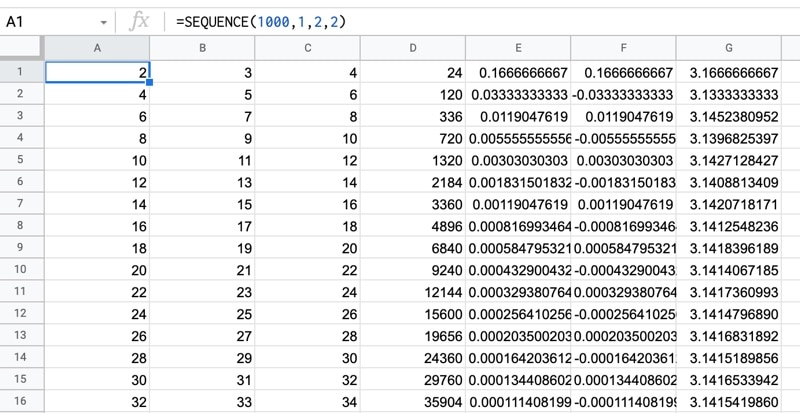# PI Function in Google Sheets And Other Fun π Facts

The PI function in Google Sheets returns the value of the mathematical constant pi (π) to 9 decimal places: 3.141592654

Pi is defined as the ratio of a circle’s circumference to its diameter.

Pi is denoted by the Greek lowercase letter π

## PI Function in Google Sheets: Syntax

`=PI()`

There are no arguments. If you put anything between the brackets the formula will give you an error.

## Circle Calculations With The PI Function

If you know the circumference of a circle then you can calculate the diameter and vice versa.

### How to calculate the circumference

Suppose you have a diameter of 10 (the units are irrelevant).

Then you calculate the circumference with this formula: the diameter multiplied by pi.

`= 10 * PI()`

### How to calculate the diameter

Now suppose you know the circumference is value 10.

The diameter is calculated as the circumference divided by pi:

`= 10 / PI()`

### How to calculate the area of a circle

The formula for the area of a circle is π * r² (pi times the radius squared).

To calculate the radius, you must divide the diameter by 2.

So, knowing the diameter d, you can calculate the area of a circle as follows: π * (d/2)²

Taking a diameter of 10, the area is:

`= PI() * ( 10 / 2 )^2`

## Creating the PI Symbol with the CHAR Function

The amazing CHAR function, which converts numbers into characters per the Unicode table, can output the symbol for pi in your Google Sheet.

Use this formula to output the pi symbol π:

`=CHAR(960)`

Setting the cell to the Merriweather font gives it the nice rounded appearance shown in this image:By the way, this formula:

`=CHAR(129383)`

will output an image of an entirely different type of pie in your Google Sheet:## PI function template

Feel free to make a copy: File > Make a copy…

If you can’t access the template, it might be because of your organization’s Google Workspace settings. If you click the link and open it in an Incognito window you’ll be able to see it.

## Approximating Pi in Google Sheets With the Nilakantha Series

The Nilakantha series, named after the 15th century mathematician of the same name, is an infinite series that converges on pi as the number of iterations rises. It converges quickly so it’s a good algorithm to use to compute the digits of pi.

The Nilakantha series is:

Let’s build an approximation of pi in a Google Sheet using this series.

### Nilakantha Series in Google Sheets With The PI Function

In cell A1, enter this formula with the SEQUENCE function:

`=SEQUENCE(1000,1,2,2)`

And in B1:

`=SEQUENCE(1000,1,3,2)`

Then in C1:

`=SEQUENCE(1000,1,4,2)`

These three formulas give us three columns of numbers with 1,000 digits each. They start with 2, 3 and 4 respectively and increment by 2.

Look closely at the infinite series definition above and you’ll notice that each row represents a denominator for each iteration of the series.

In cell D1, multiply the values together for each row:

`=PRODUCT(A1:C1)`

Copy this formula down the column.

This formula generates the denominators for the series.

In cell E1, put this product as the denominator to calculate the series values:

`= 4 / D1`

Next, we need to alternate adding and subtracting these values, which we can do with this formula:

`=IF( ISODD( ROW() ), E1 , UMINUS( E1 ) )`

This IF formula checks if we’re on an odd numbered row (e.g. row 1) using the ISODD function and, if we are, returns the number unchanged. But if we’re on an even numbered row, it returns the negative value from column E, using the UMINUS function.

Finally, we can approximate pi in column G.

In G1, put this formula to start the series:

`= 3 + F1`

In cell G2, put this formula:

`= G1 + F2`

and drag this formula all the way to the bottom of the range.

With 1,000 rows, we get a value for pi of 3.1415926533, which is a similar level of accuracy to the PI function.

Here are the first 16 iterations, the remaining rows up to 1,000 can be found in the template linked above in this article:Now, you might be wondering how many digits of pi have been calculated…

…well it’s a whopping 31.4 trillion digits!

Obviously, that is not a calculation you can perform in your Google Sheet, but interestingly, it was performed on Google Cloud infrastructure!

Update: The record is now 50 trillion digits, broken by an enthusiast with a self-built server setup. Super impressive!

## Getting More Digits of Pi in Google Sheets

Whilst we can’t get 50 trillion digits of pi into a Google Sheet, we can call the Pi Delivery API and retrieve more digits.

Here’s the code to request the first 1,000 digits of pi and display them in a Google Sheet as a text string (because numbers get truncated):

```/**
* function to call the Pi API
* https://pi.delivery/#apipi_get
*/
function getPI() {

// endpoint
const root = 'https://api.pi.delivery/v1/pi';

// start point and number of digits
const startDigit = 0;
const numDigits = 1000; // max request is 1000 digits in 1 call

// create endpoint
const endpoint = root + '?start=' + startDigit + '&numberOfDigits=' + numDigits;

// call the PI API to fetch digits of pi
const response = UrlFetchApp.fetch(endpoint);
const result = JSON.parse(response.getContentText());

// get pi
// keep pi as a string otherwise it gets truncated if a number
// add single tick in front of the 3 to avoid automatic conversion to number in Sheet
const piDigits = result.content;
const pi = "'3." + piDigits.substring(1);

// paste result into Google Sheet